# Math in Focus Grade 4 Chapter 4 Practice 2 Answer Key Using a Table

Go through the Math in Focus Grade 4 Workbook Answer Key Chapter 4 Practice 2 Using a Table to finish your assignments.

## Math in Focus Grade 4 Chapter 4 Practice 2 Answer Key Using a Table

Use the data in the table to complete the sentences below.

The table shows pictures at rows, columns, and intersections.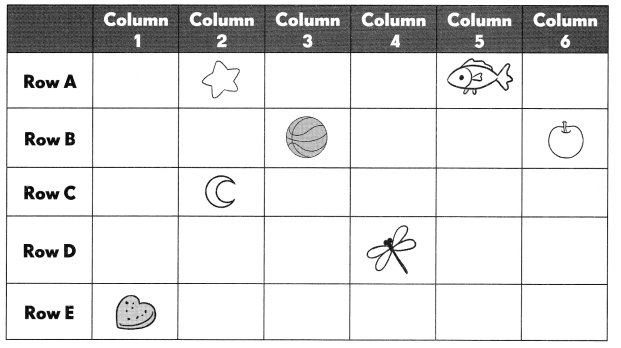Example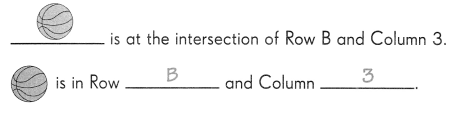Question 1.
___________ is at the intersection of Row E and Column 1.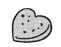is at the intersection of Row E and Column 1.

Question 2.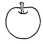is in Row __________ and Column ____.is in Row B and Column 6.

Question 3.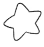is in Row __________ and Column __________is in Row A and Column 2.

Question 4.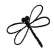is in Row __________ and Column __________is in Row D and Column 4.

The table shows part of Bill’s class schedule from Monday through Wednesday.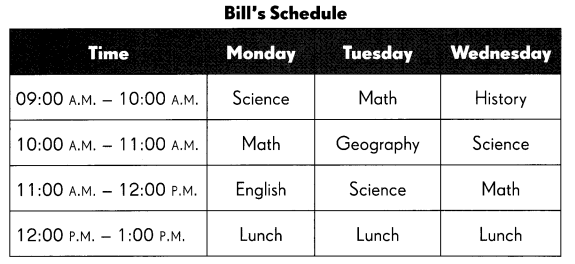Use the table to answer the questions.

Question 5.
What class does Bill have between 10:00 A.M. and 11:00 A.M. on Mondays? __________
Math.

Explanation:
Bill has Math class between 10:00 A.M. and 11:00 A.M. on Mondays

Question 6.
What class does Bill have between 9:00 A.M. and 10:00 A.M. on Wednesdays? ____
History.

Explanation:
Bill has history class between 9:00 A.M. and 10:00 A.M. on Wednesdays

Question 7.
His lunch break on Wednesday is between ____________________
12:00 P.M to 1:P.M.

Explanation:
Bill’s lunch break on Wednesday is between 12:00 P.M to 1:P.M.

Question 8.
His Math class on _________________ is between 11:00 A.M. and 1 2:00 P.M.
Wednesday.

Explanation:
His Math class on Wednesday is between 11:00 A.M. and 1 2:00 P.M.

Question 9.
His Geography class on ________________ is between 10:00 A.M. and 11:00 A.M.
Tuesday.

Explanation:
His Geography class on Tuesday is between 10:00 A.M. and 11:00 A.M.

Maria and Vinny collected stamps from three different countries: Singapore, Malaysia, and Thailand. The number of stamps collected is shown in the table below.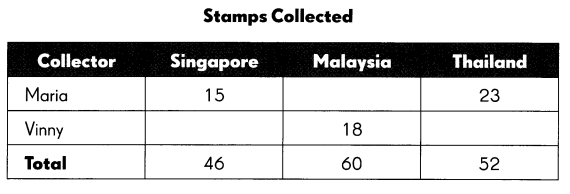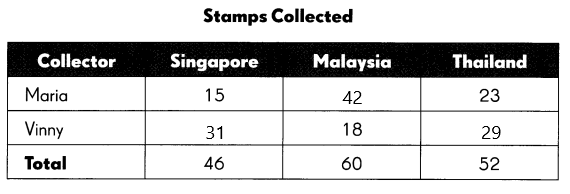Complete the table, and answer the questions.

Question 10.
How many Thailand stamps did Vinny collect? ____
29 Stamps.

Explanation:
Vinny collected 52-23 which is 29 Thailand stamps.

Question 11.
How many Thailand stamps did Maria and Vinny collect altogether? ____
52 stamps.

Explanation:
The total number of Thailand stamps Maria and Vinny collect altogether is 23+29 which is 52 stamps.

Question 12.
How many more Malaysia stamps than Singapore stamps did Maria and Vinny collect altogether? ____
14 more stamps.

Explanation:
The number of Malaysia stamps did Maria and Vinny collect altogether is 60 stamps and the number of Singapore stamps did Maria and Vinny collect altogether is 46 stamps. So the number of many Malaysia stamps than Singapore stamps is 60-46 which is 14 stamps.

Question 13.
Who collected more stamps: Maria or Vinny? ____
Maria.

Explanation:
The total number of stamps did Maria collected is 15+42+43 which is 80 stamps and Vinny collected 31+18+29 which is 78 stamps. So Maria collected more stamps.

Question 14.
How many stamps did they collect altogether? ____
158 stamps.

Explanation:
The number of stamps did they collect altogether is 78+80 which is 158 stamps.

The table shows the number of quarters and nickels that five students saved.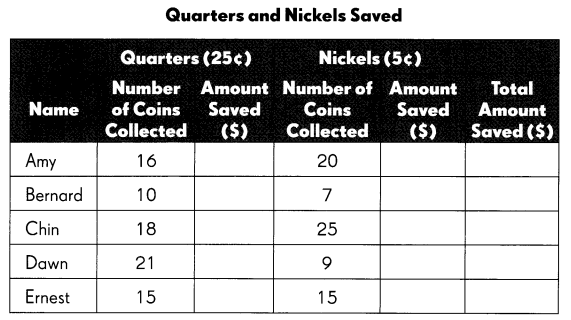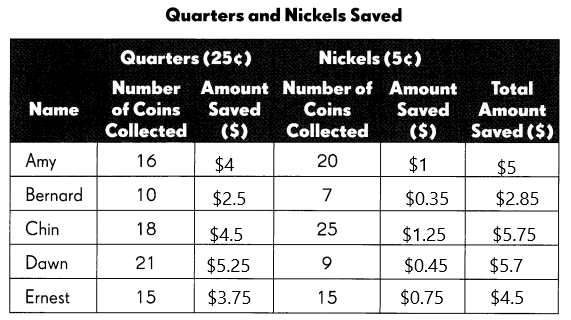Complete the table, and answer the questions.

Question 15.
Who saved the greatest amount? ____
Chin.

Explanation:
The greatest amount saved by Chin.

Question 16.
Who saved the least amount? ____
$2.85. Explanation: The least amount saved is$2.85.

Question 17.
How many more coins did Chin collect than Ernest? ____
13 more coins did Chin collect than Ernest.

Explanation:
The total number of coins collected by Chin is 43 and the total number of coins collected by Ernest is 30. So there are 43-30 which is 13 more coins did Chin collect than Ernest.

Question 18.
How much more must Bernard save in order to have the same amount as Dawn? ____
Bernard needs to save $2.85. Explanation: The total amount did Bernard have is$2.85 and the total amount did Dawn have is $5.7. So Bernard needs to save$5.7-$2.85 which is$2.85.
Which two students saved a total of less than $7.50? How much less ? ____ Answer: Bernard and Ernest. Explanation: The two students who saved a total of less than$7.50 is Bernard and Ernest which is $2.85+$4.5 which is $7.35. Question 20. Which two students collected the same number of coins? Answer: Dawn and Ernest. Explanation: Dawn and Ernest are the two students who collected the same number of coins. Question 21. Of the two students in Exercise 20, who saved more money? How much more? Answer: Chin$5.75.